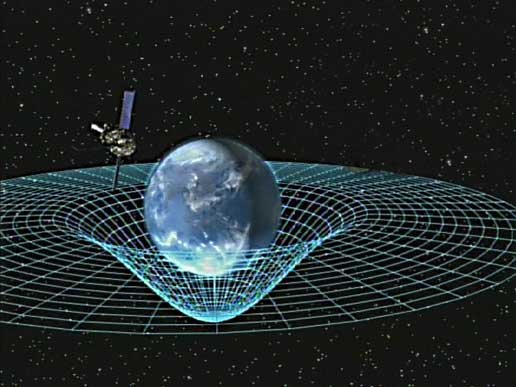# The Field Equations of General Relativity# Keeping It Relatively Simple

The Einstein Field Equations of General Relativity are vast and complex, but they can be written with deceptive simplicity.  Using modern notation, the field equations can be formulated as$\boldsymbol G = \frac {- 8 \pi G}{c^4} \boldsymbol T$   Continue reading The Field Equations of General Relativity